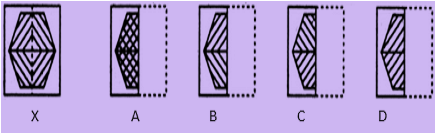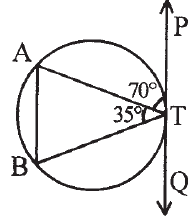# SOF - IMO PDF Sample Papers for Class 10

Class 10 sample paper & practice questions for International Mathematics Olympiad (IMO) level 1 are given below. Syllabus for level 1 is also mentioned for these exams. You can refer these sample paper & quiz for preparing for the exam.#### Resources:

##### Sample Questions from Olympiad Success:
 Q.1 Q.2 Q.3 Q.4 Q.5 Q.6 Q.7 Q.8 Q.9 Q.10
 Q.1 Find out from the four alternatives as to how the pattern would appear when the transparent sheet is folded at the dotted line.a) B b) D c) C d) A
 Q.2 6x – 3y + 10 = 0, 2x – y + 9 = 0 represents two lines which are: a) Intersecting at exactly one point b) Intersecting at exactly two points c) Coincident d) Parallel
 Q.3 Look at the image given below and find the value of ∠BTQ.a) 30⁰ b) 45⁰ c) 75⁰ d) 90⁰
 Q.4 A jar containing a mixture of milk and water has 55% milk. A part of this mixture is replaced by another mixture of milk and water having 20% milk. If now the mixture has 35% milk, what fraction of the jar was not replaced? a) 3/4 b) 4/7 c) 3/7 d) 4/3
 Q.5 Find the value of sin² 10⁰ + sin² 80⁰: a) 0 b) 1 c) 2 d) 3
 Q.6 A tangent to a circle (centre O) drawn from an exterior point T to the circle and touches the circle at a point P on the circumference. Which of the following statement is TRUE? a) ∠TPO > 90° b) ∠TPO < 180° c) ∠TPO < 90° d) ∠TPO = 90°
 Q.7 If you stand at the centre, and face towards South. Now you move at an angle of 100 degrees clockwise, then in which direction do you face now? a) North b) South c) North-West d) South-West
 Q.8 If 4th and 8th terms of an A.P. are 11 and 23 respectively, find a and d. a) a = 2, d = 3 b) a = 2, d = 2 c) a = 3, d = 3 d) a = 1, d = 3
 Q.9 What will be the remainder if f(x) = x³ + 4x² – 3x + 1 is divided by (x – 2)? a) 18 b) 20 c) 19 d) 31
 Q.10 T has child J. J has children M and N. M is married to C and has a child O. What is the relation between O and T? a) mother-in-law b) father-in-law c) grandparent d) great grandparentSample PDF of SOF - International Mathematics Olympiad (IMO) PDF Sample Papers for Class 10:

 Q.1 )a Q.2 )d Q.3 )c Q.4 )a Q.5 )b Q.6 )d Q.7 )c Q.8 )a Q.9 )c Q.10 )d

Q.1 : a | Q.2 : d | Q.3 : c | Q.4 : a | Q.5 : b | Q.6 : d | Q.7 : c | Q.8 : a | Q.9 : c | Q.10 : d